### Objective

Readers will be able to use the ARRAYFORMULA(), VLOOKUP() and MATCH() functions in Google Sheets to combine multiple sources of data on to a single sheet.

### Why this matters

Schools may have multiple core data systems with limited integration. Getting an accurate understanding of a child's progress towards key benchmarks can make taming lions look like a walk in the park. For many educators, getting attendance, testing, course performance, and behavioral data for one student means logging into up to four different systems and a lot of screen real estate. In order to get a complete picture of a student's performance, it is important to be able to aggregate data from multiple systems into one view.

### Prerequisite knowledge

Importing data into Google Spreadsheets, creating a Google Spreadsheet, navigating a Google Spreadsheet, basic understanding of how spreadsheet functions work.

### Introduction

In this tutorial we will use three Google Spreadsheet functions to robustly combine data from multiple child sheets into a single master sheet. First I will describe the initial setup of a Google Spreadsheet containing multiple data sheets.  Second, I will introduce MATCH() and VLOOKUP(), their syntax, purpose, and examples of their use. Next, I will describe how to combine MATCH() and VLOOKUP(). After that, I will introduce ARRAYFORMULA() and in the end, I will describe how to combine the three functions to create a single sheet view of all the disparate data. One view to rule them all, as the saying goes.

### Initial Setup

1. Create a new Google Spreadsheet.
2. Use the import CSV feature in the "File" menu to upload multiple data sets, such as test scores, attendance, behavior data, etc. All data must have a common unique identifier associated with each record. In this case, each student has a unique student identification number. The unique identifier must be in a column to the left of the data that will be aggregated from each import.
3. Create a parent sheet in the Google Spreadsheet, which we will call "Student Data".
4. Create a student record list by pasting a complete list of student IDs, first names, and lastnames into the first columns of the "Student Data" sheet with clearly titled column headers. While this example uses student records, clients, customers, vendors, etc. could be used as records to aggregate data on as well.
5. Title columns to the right of the initial student record list. In this example we will aggregate student GPA, attendance, and standardized test scores. The titles of each column must match the titles of the columns in the child sheets containing the imported data from step 2. For example, if the attendance child sheet contains a column header called "Year to Date Att" that I want to include in the "Student Data" sheet, I will create a column header in the "Student Data" sheet called "Year to Date Att".

Once the spreadsheet child sheets are all imported and the master "Student Data" sheet contains a row for each record and column headers that match desired data from the child data sheets, we are ready to move on and use the MATCH(), VLOOKUP(), and ARRAYFORMULA() functions to aggregate child sheet data on the "Student Data" sheet.

You can make a copy of a pre-built demo spreadsheet with all of the above steps already accomplished here.

### Match()

Arguments: MATCH(search_key, range, [search_type])

Summary: Returns the relative position of an item in a range that matches a specified value.

search_key: The value to search for.

range: The one-dimensional array to be searched. Or, more simply a list of items.

search_type: The manner in which to search. In 99% of use cases for this function, I've always needed an exact match, so the search type is 0 or FALSE.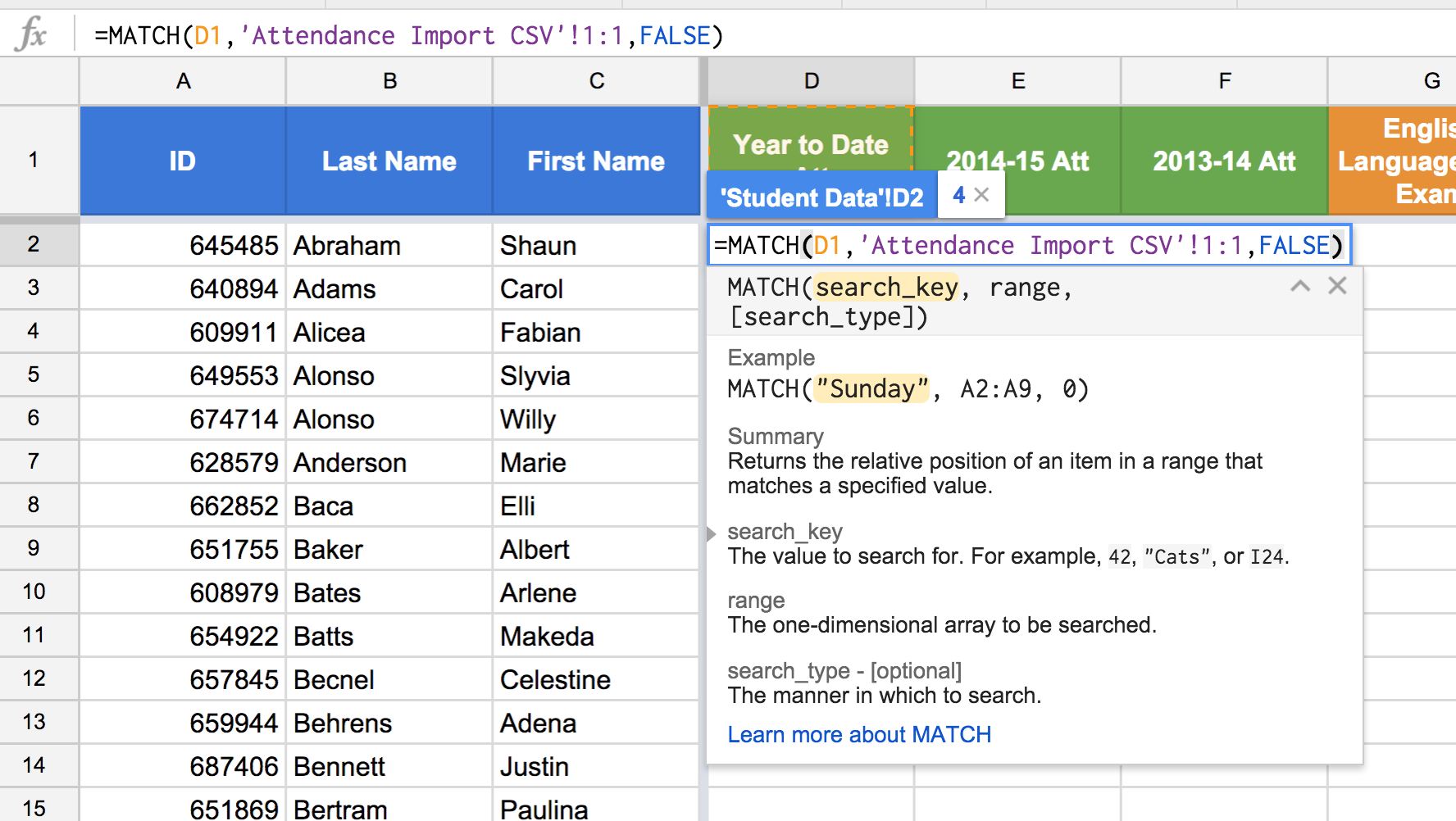Figure 1: Using MATCH() to return the relative position of the contents of cell D1, "Year to Date Att", in the range 'Attendance Import CSV'!1:1, the first row of the Attendance Import CSV child sheet, using an exact match (false).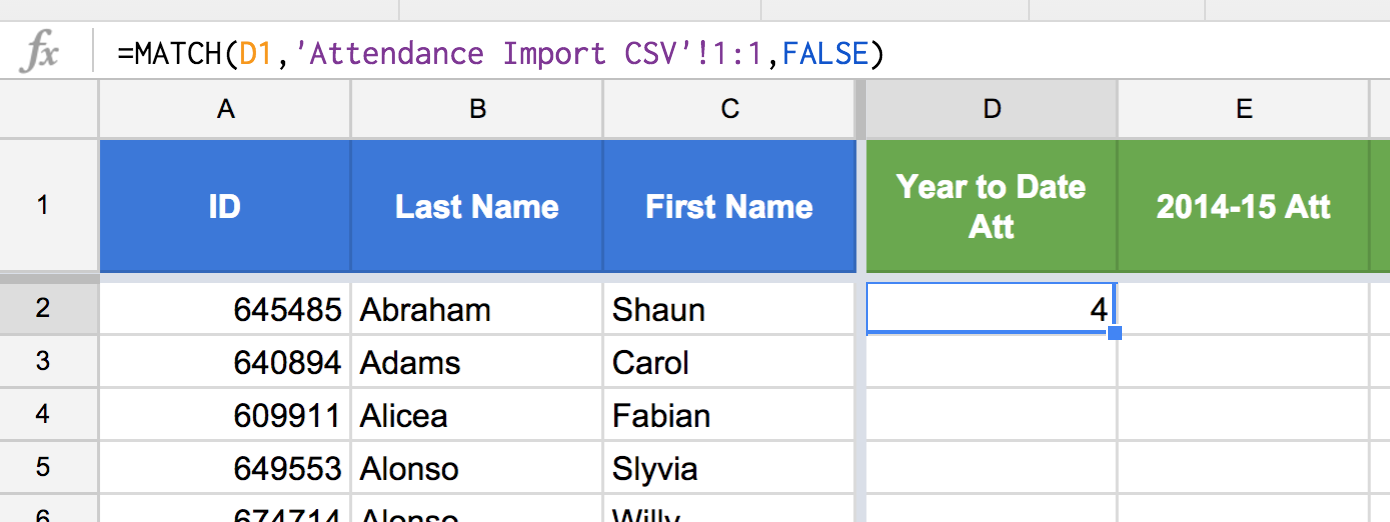Figure 2: The MATCH() in Figure 1 returns a 4, which is the relative position of the search key, "Year to Date Att" in the range 'Attendance Import CSV'!1:1, the first row of the Attendance Import CSV child sheet.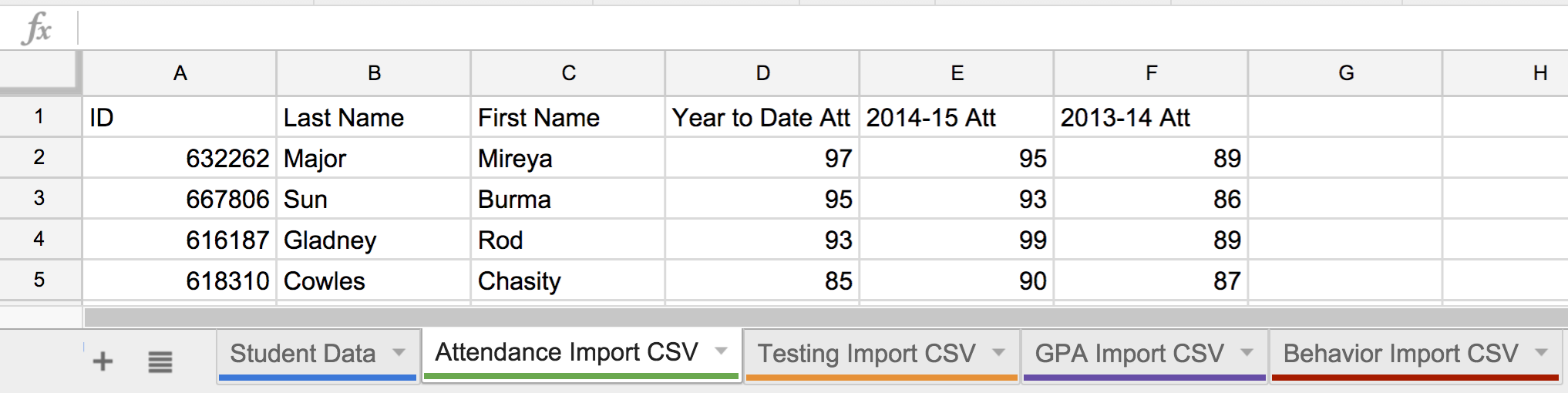Figure 3: Notice that "Year to Date Att" is the fourth value in the one-dimensional range in row 1 of the Attendance Import CSV child sheet. In other words, starting from the left, "ID" is the first value in row 1, "Last Name" is the second value, "First Name" is the third value, and so on. Match() tells us where in a one-dimensional range an item is located.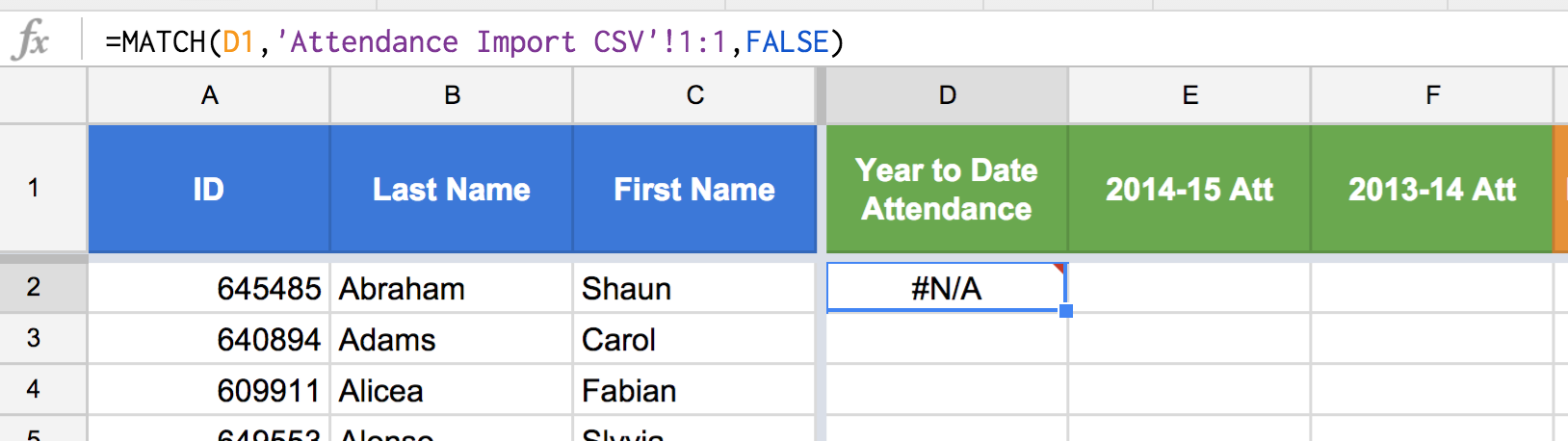Figure 4: A common mistake is attempting to match a search_key that is not actually in the range used in argument two. In the above case we get "#N/A" because "Year to Date Attendance", the search key, is not in the first row of the Attendance Import CSV. The key point is to be very careful that you are trying to match items that are exactly the same in the search_key and the range.

### VLOOKUP

Arguments: VLOOKUP(search_key, range, index, [is_sorted])

Summary: Vertical lookup. Searches down the first column of a range for a key and returns the value of a specified cell in the row found.

search_key: The value to search for.

range: The range to consider for the search. The first column in the range is searched for the key and returns the value of a specified cell in the row found.

index: The column index of the value to be returned, where the first column in range is numbered 1.

is_sorted: Indicates whether the column to be searched (the first column of the specified range) is sorted, in which case the closest match for search_key will be returned. As is the case with MATCH(), 99% of the times I've used VLOOKUP(), the is_sorted argument should be FALSE because I am interested in an exact match.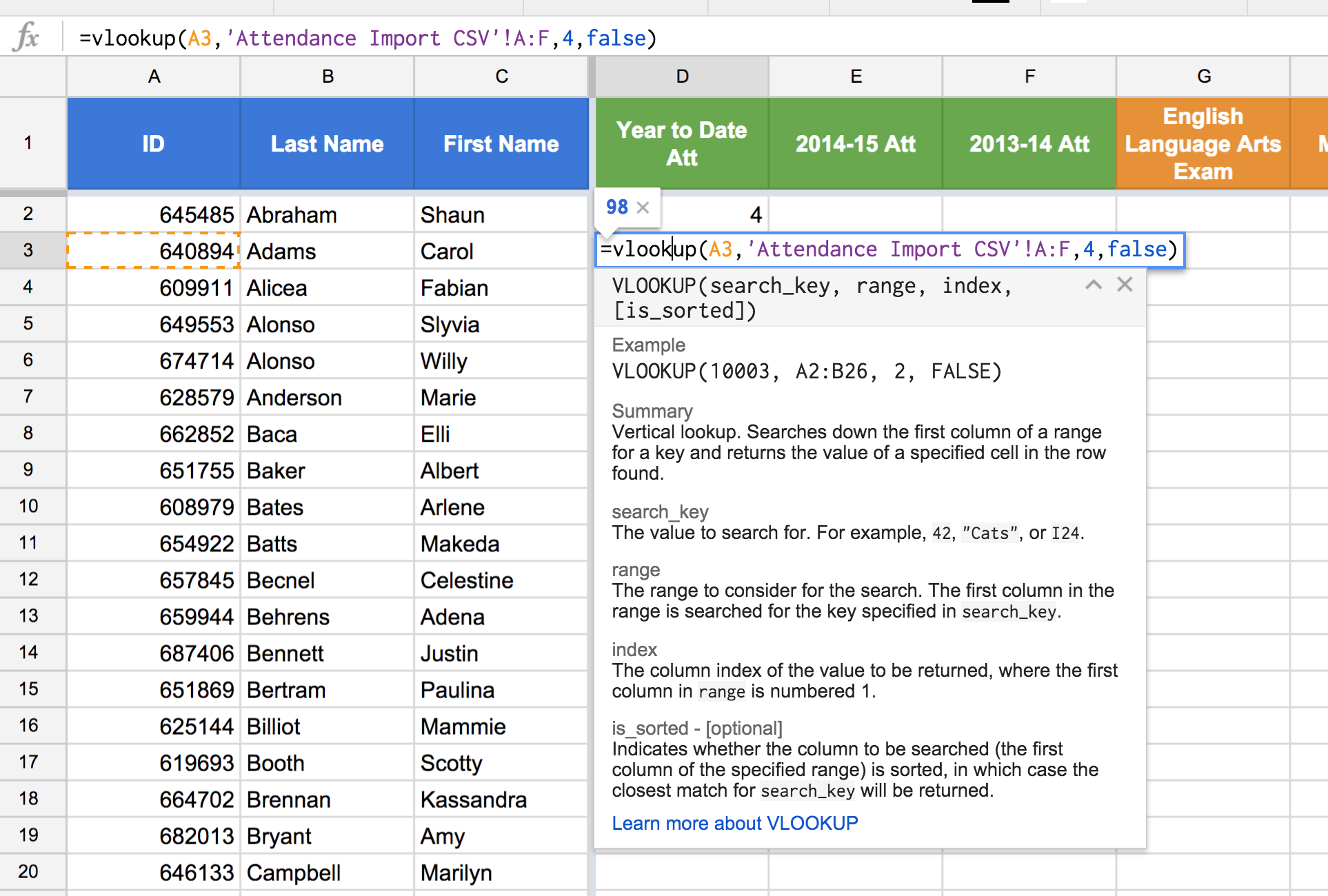Figure 5: We are looking up Carol Adams's Year to Date Attendance in the "Attendance Import CSV" child sheet by using her student ID as a search_key. More specifically, we are using VLOOKUP() to return the value in the fourth column of the range 'Attendance Import CSV'!A:F, where the student id "640894", cell A3, is found in the first column of the  range 'Attendance Import CSV'!A:F. Since we are only interested in the exact student ID match, we use FALSE for the optional "is_sorted" argument.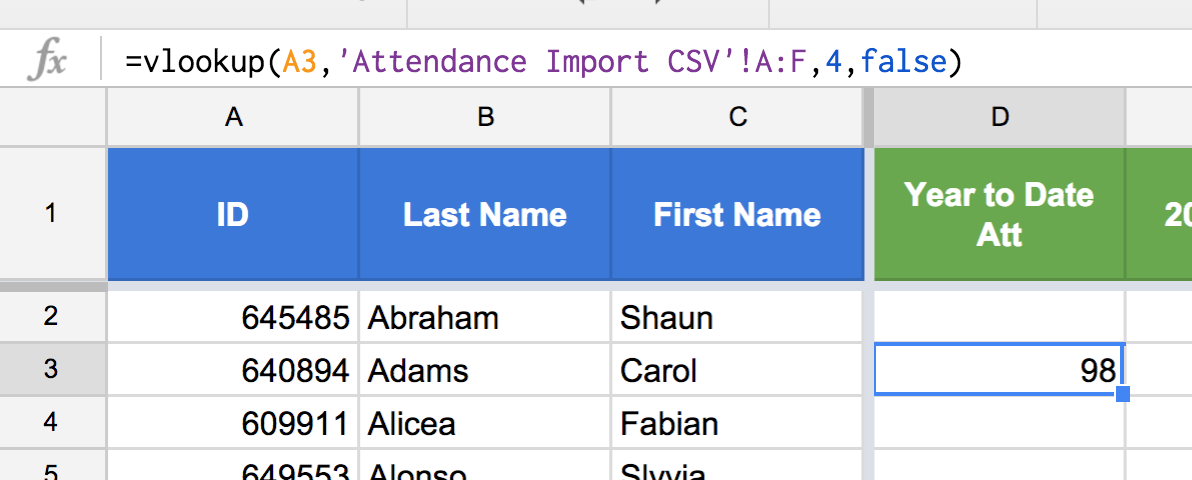Figure 6: Carol Adams's Year to Date Attendance value on the Attendance Import CSV child sheet is 98.

For additional help with VLOOKUP, check out this handy CloudLab New Visions tutorial that I co-authored in another lifetime.

### Combining MATCH() and VLOOKUP()

Think back to the MATCH() example above which returned a 4 to indicate the relative position of "Year to Date Att" in the first row of the Attendance Import CSV child sheet. Perhaps you have already realized that the return value for our MATCH() function is the same value as the index argument in our VLOOKUP() example. This was not a coincidence and herein lies the beauty and elegance of MATCH() and VLOOKUP().

To combine MATCH() and VLOOKUP, simply replace the index argument in VLOOKUP() with the entire MATCH() function.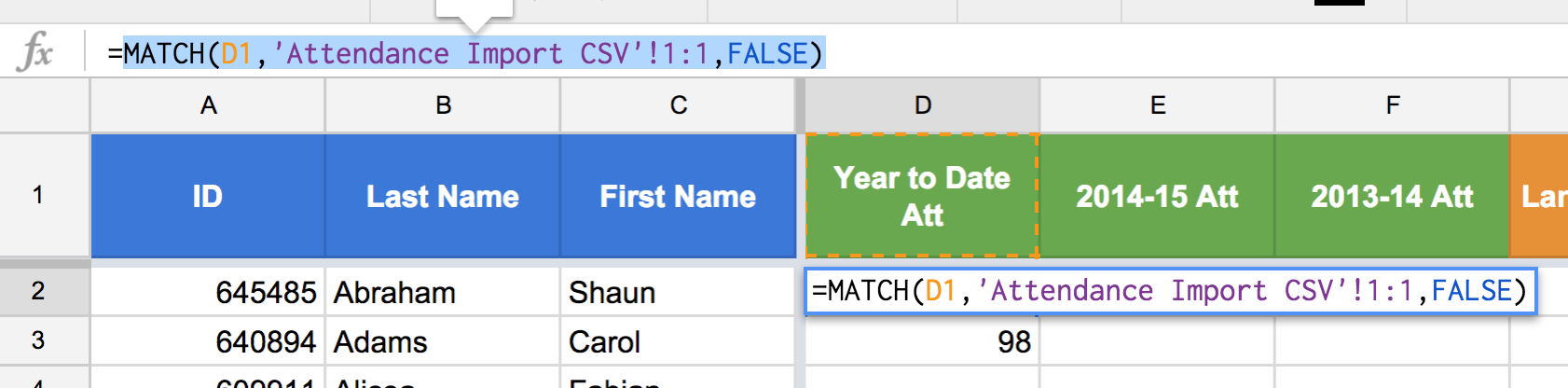Figure 7: Highlight the MATCH() function, without the "=" sign. Use ctrl+C or cmd+C to copy the text of the function.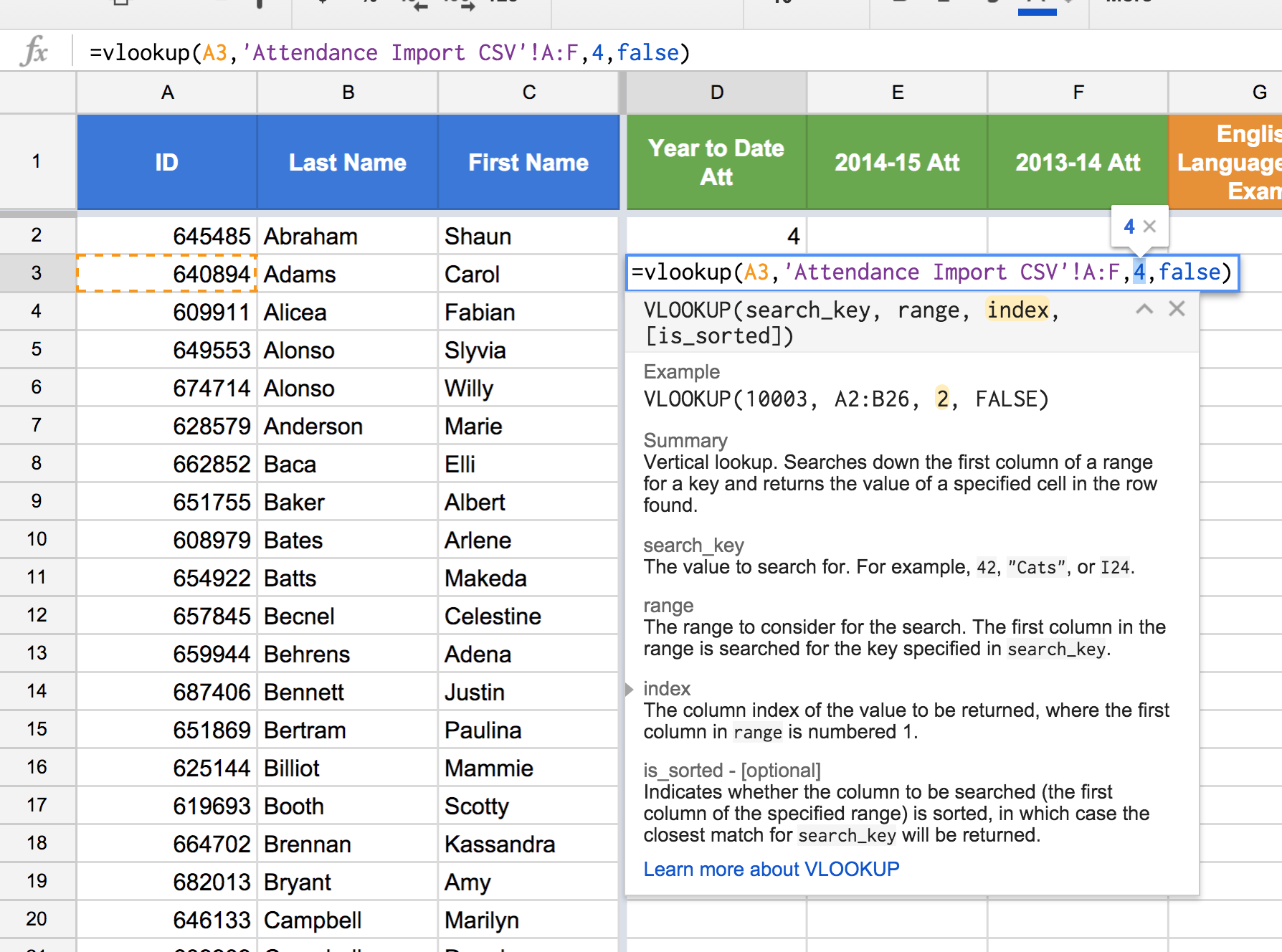Figure 8: Highlight the index argument, which is 4, in the VLOOKUP() function. Use ctrl+V or cmd+V to paste the MATCH() function as the index argument.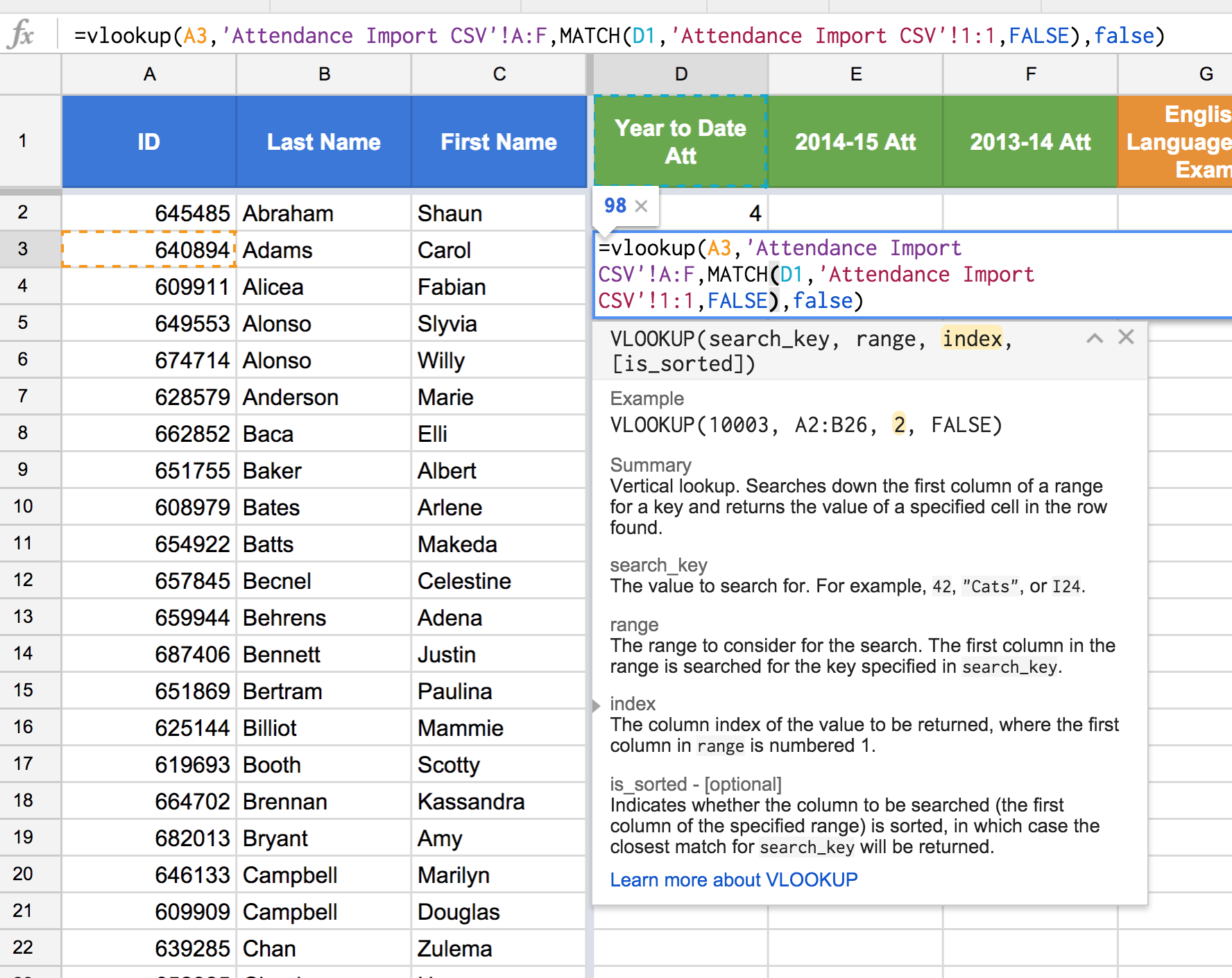Figure 9: Using the MATCH() function as the index argument in VLOOKUP(). Since the MATCH() function returns a 4, the value returned by the VLOOKUP() will remain, 98, which is the value in the fourth column of the Attendance Import CSV child sheet and the row matching Carol Adams's student ID.

### ArrayFormula

Arguments: ARRAYFORMULA(array_formula)

Summary: Enables the display of values returned from an array formula into multiple rows and/or columns and the use of non-array functions with arrays.

array_formula: A range, mathematical expression using one cell range or multiple ranges of the same size, or a function that returns a result greater than one cell.

Disclaimer: Arrayformula is very conceptually challenging to use and explain. The best way to explain how we will use it in this tutorial is to say that ArrayFormula will allow us to lookup and return many values at once. VLOOKUP() is a non-array function, but by using ARRAYFORMULA() we can actually use an array as the search_key.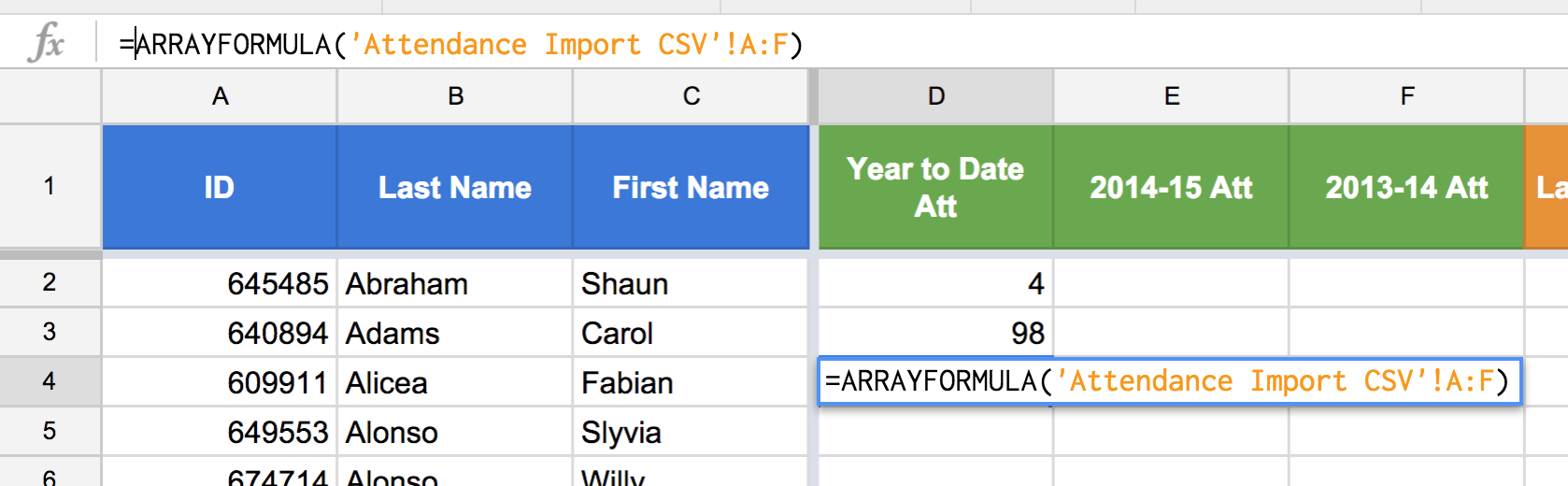Figure 10: In this example we are using ARRAYFORMULA() to return the contents of the Attendance Import CSV child sheet.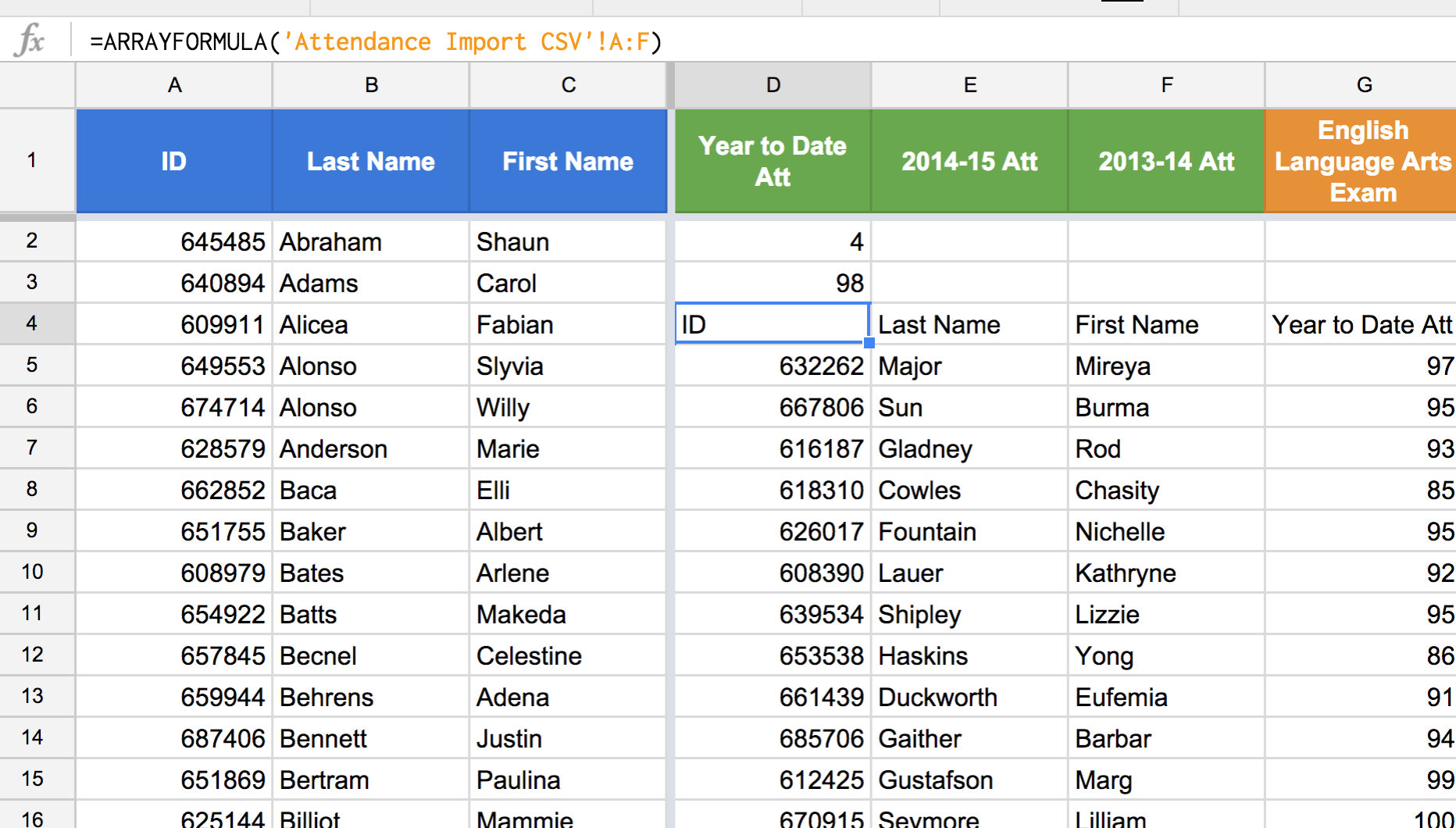Figure 11: The returned values from the Attendance Import CSV child sheet.

### Combining MATCH, VLookup, and Arrayformula

We have already been able to combine MATCH() and VLOOKUP() to find Carol Adams's year to date attendance. We next need to find the year to date attendance of all of our hypothetical students. To do so, we will wrap the entire VLOOKUP() / MATCH() combination in an ARRAYFORMULA() and we will change the first argument of the VLOOKUP() from A3, Carol Adams's student ID, to A2:A, which is the range containing all of our student IDs.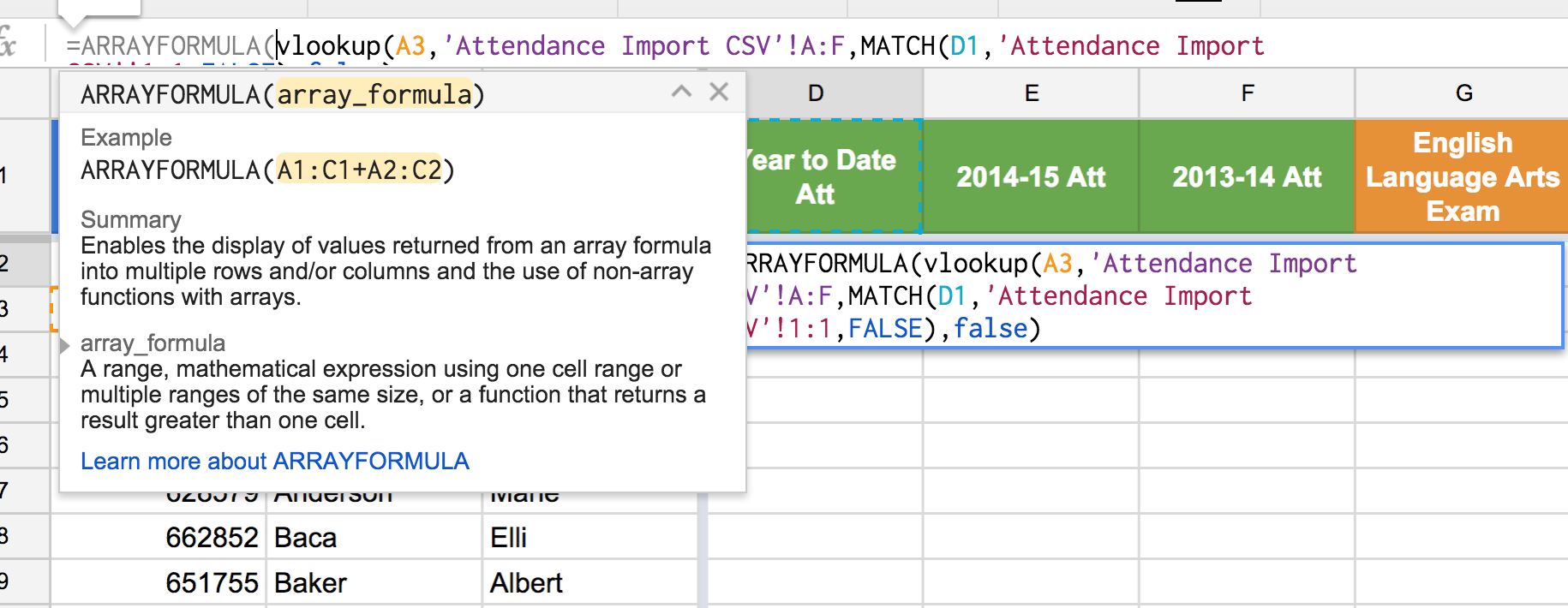Figure 12: Insert "ARRAYFORMULA" and open parentheses before the start of the VLOOKUP() function. Do not worry about closing the parentheses, Sheets will do that automatically.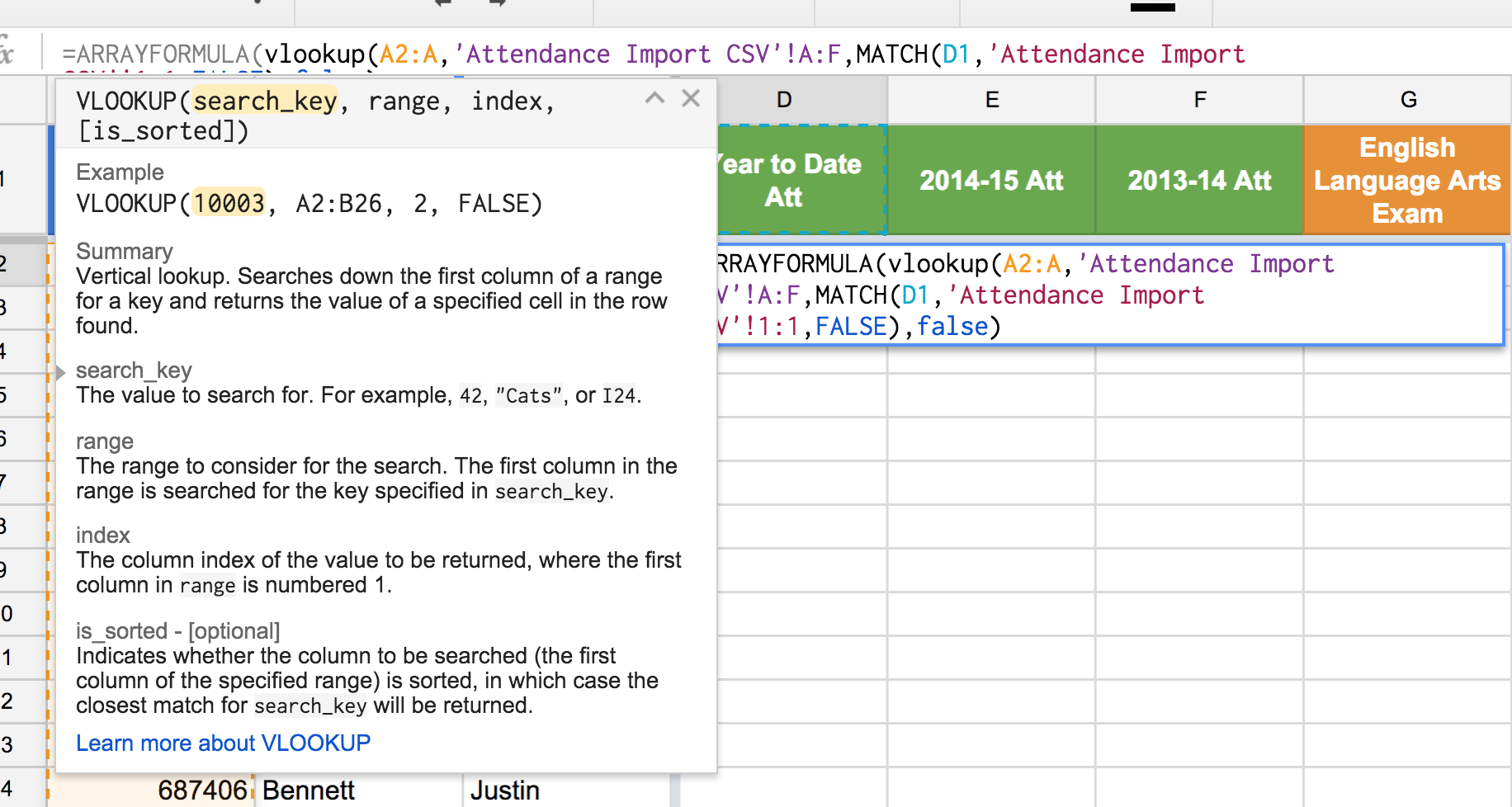Figure 13: Change the search_key argument in VLOOKUP() from A3, which is a single student ID, to A2:A, which is all of the student IDs.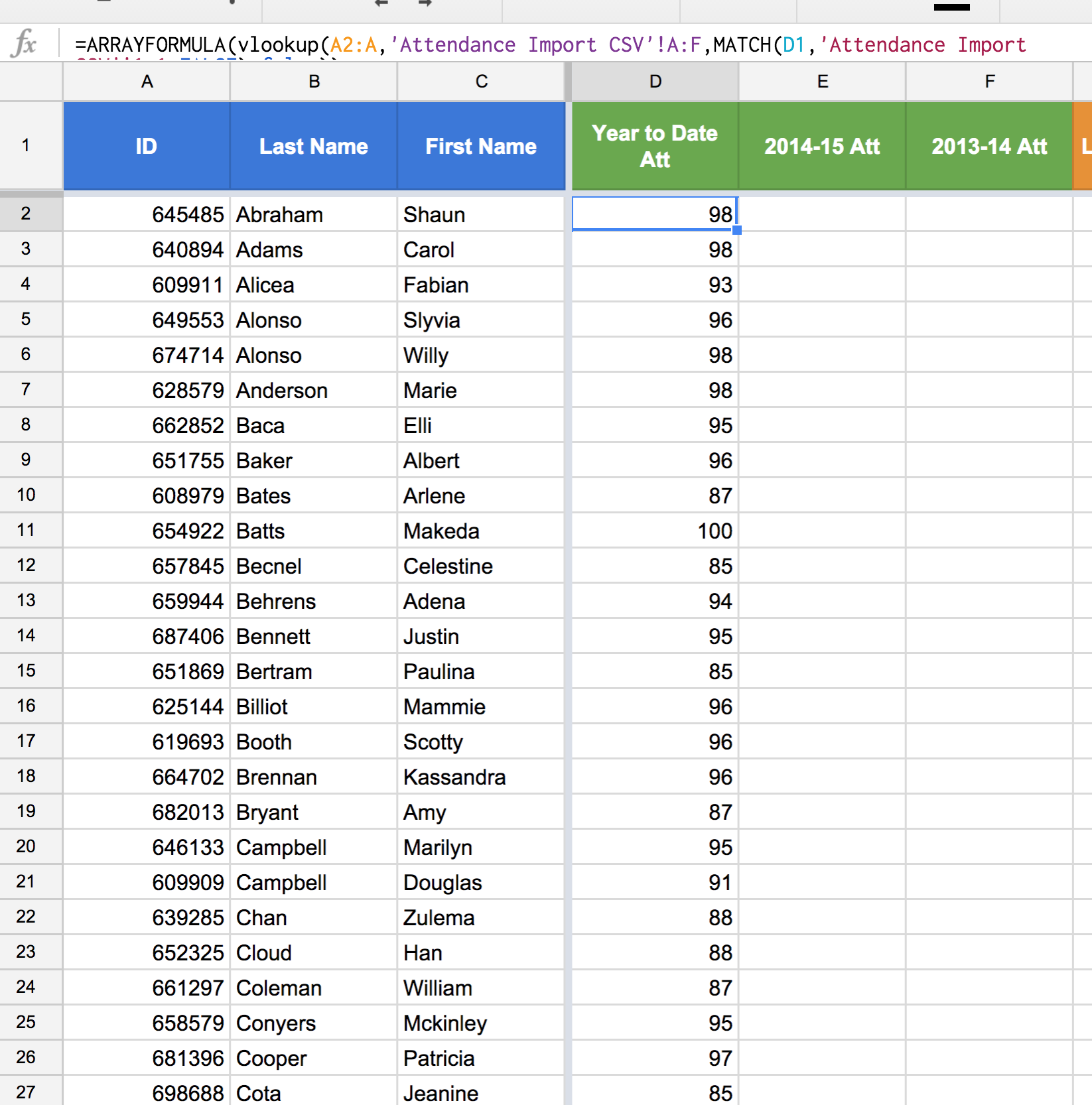Figure 14: ARRAYFORMULA() is applying the VLOOKUP() / MATCH() combination to the entire set of student IDs passed into the VLOOKUP() search_key. The result is that we are now reading in all of our students' year to date attendance values from the Attendance Import CSV child sheet.

### STatic References

The next step is to use the "\$" in our formula to keep certain references static so that we can copy and paste this formula and continue to reap the rewards of its power.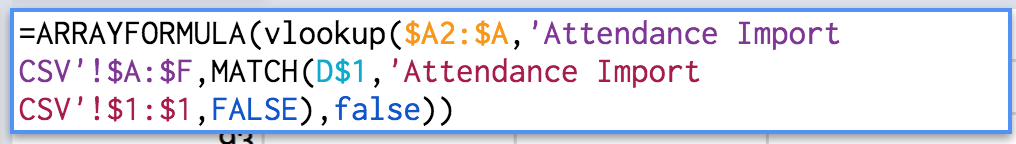Figure 15: The static references we need to apply to fully reap the rewards of the power of this triumvirate.
• Change A2:A to \$A2:\$A because as we start pasting this formula to the right, we want the search_key to remain the student IDs in column A.
• Change A:F to \$A:\$F because we want the range argument in VLOOKUP() to stay in the same place. If we forget this step, we might accidentally shift the VLOOKUP() range such that it no longer includes the student ID column.
• Change D1 to D\$1 because we always want the MATCH() search_key to be our column headers, but we also want to be able to shift which column header is referenced. For example, in Column E, to look up all of the 2014-15 attendance averages, we will want the search_key in the MATCH() function to be C\$1. In other words, do not put a \$ sign in front of the column reference, only the row reference.
• Change 1:1 to \$1:\$1 just for fun because we are only going to be copying and pasting the formula horizontally, which means the 1:1 will not actually shift. However, there is something to be said for consistency.

### Copying and Pasting the Triumvirate Function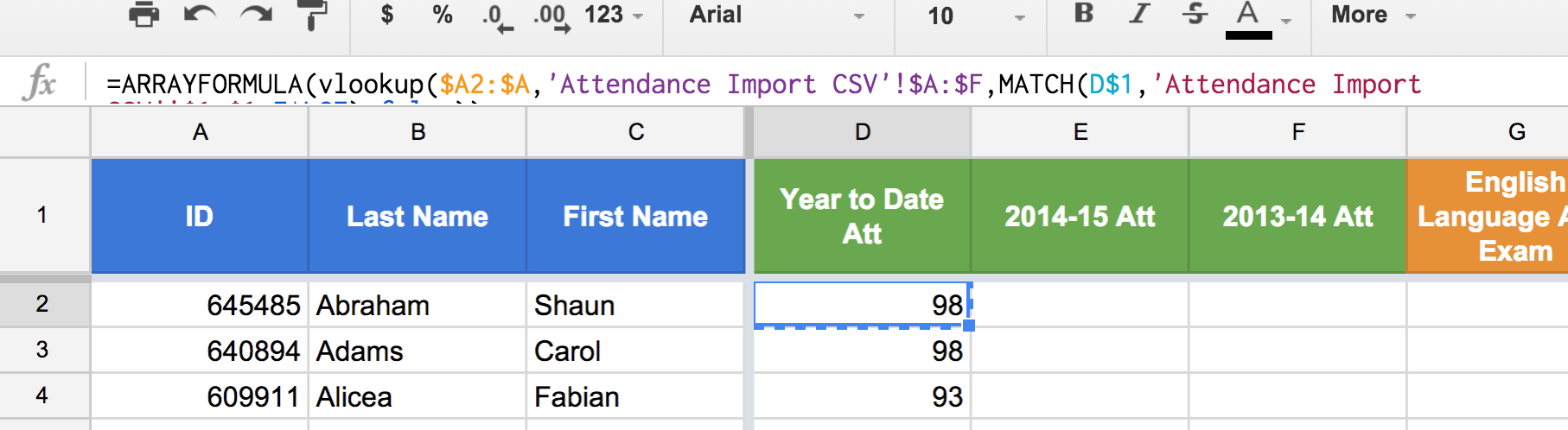Figure 16: Copy the entire formula in cell D2.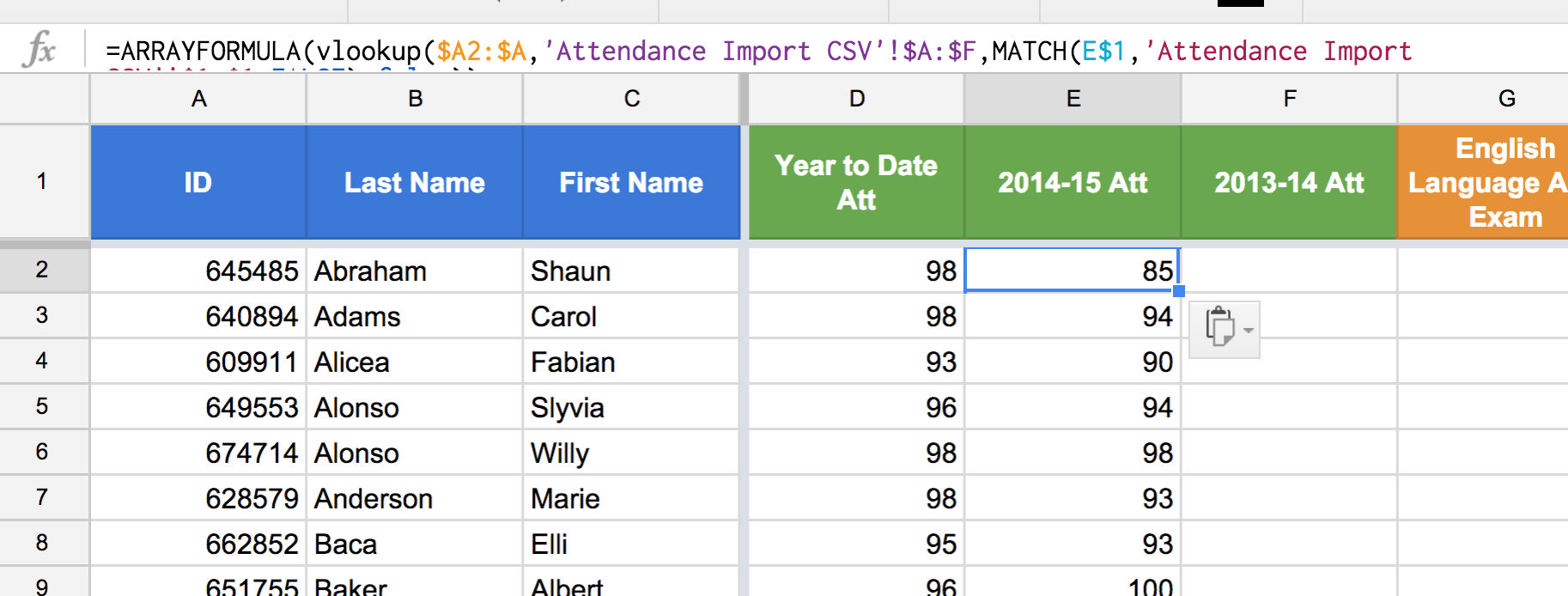Figure 17: Paste the formula from cell D2 into cell E2. Notice how the MATCH() search_key shifted from D\$1 to E\$1 while all other cell references remained static.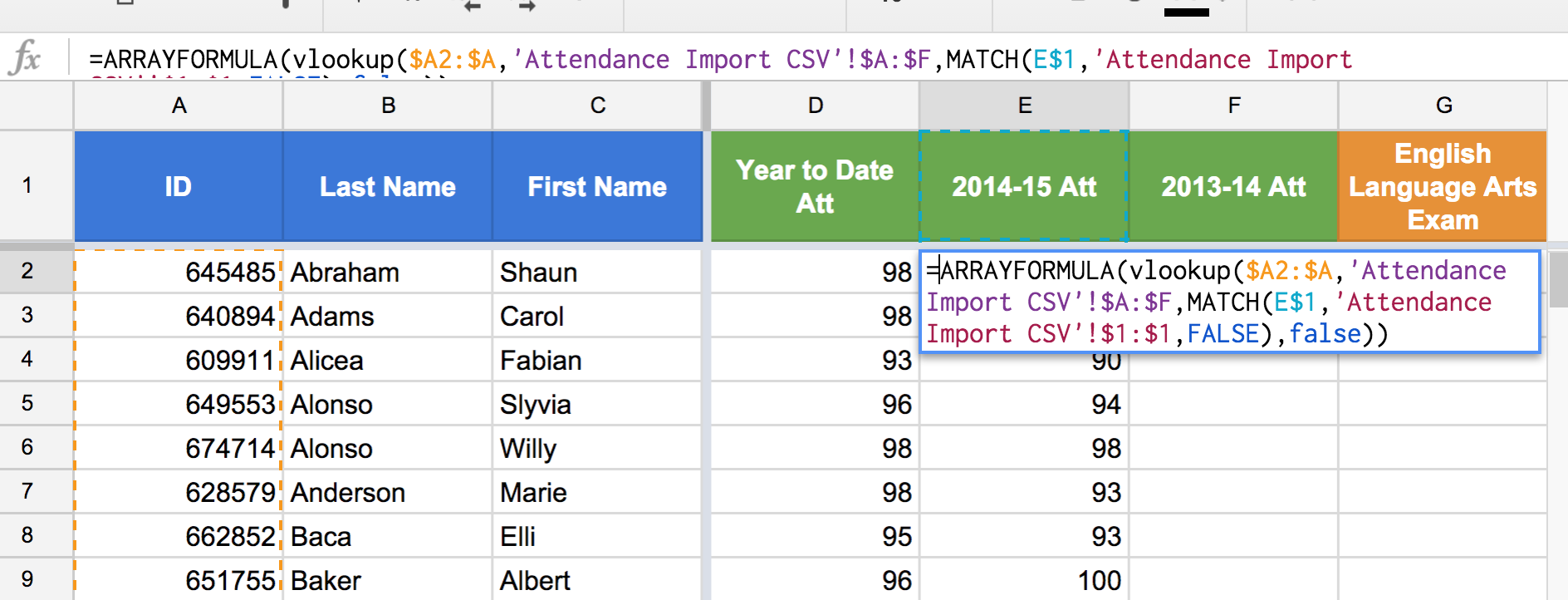Figure 18: A closer look at the effect of the copy and paste on our cell references.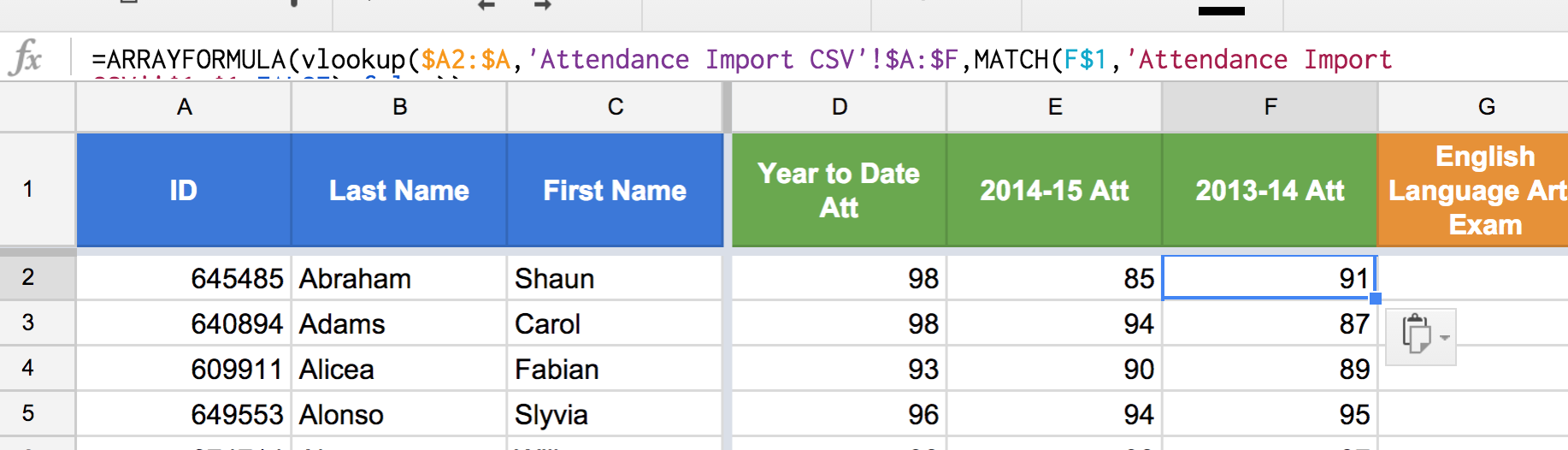Figure 19: Repeat the same copy / paste procedure for the 2013-14 Att column.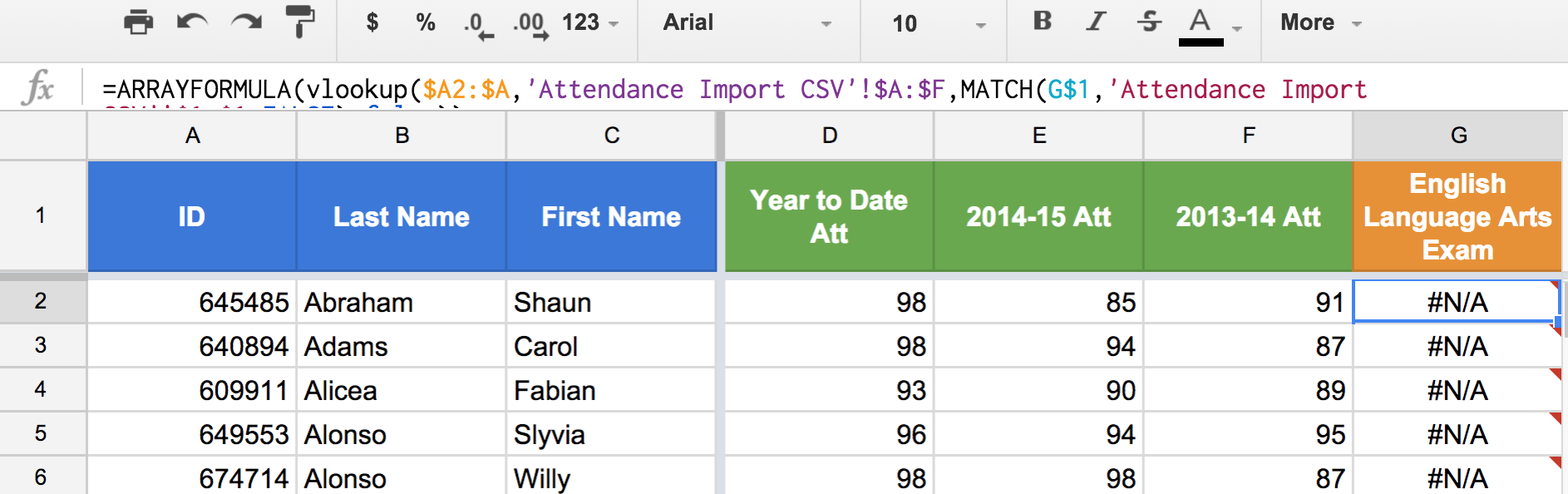Figure 20: Notice how the copy / paste procedure breaks on column G. The reason for this error is that "English Language Arts Exam" is not a value in the header row of the Attendance Import CSV child sheet. Therefore, the MATCH() function is not able to find an exact match and is returning an error.

### Changing Child Sheets

To fix the error in Figure 20, we must change the sheet being referenced in the range arguments of both VLOOKUP() and MATH().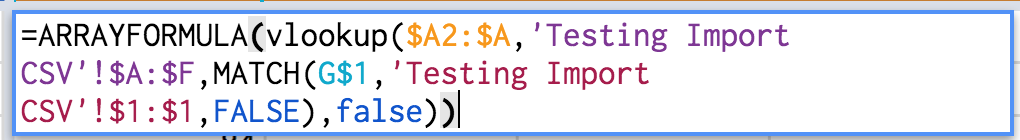Figure 21: Change the range arguments in the VLOOKUP() and MATCH() functions to reference the ranges containing the English Language Arts Exam data. In this case the child sheet is called "Testing Import CSV"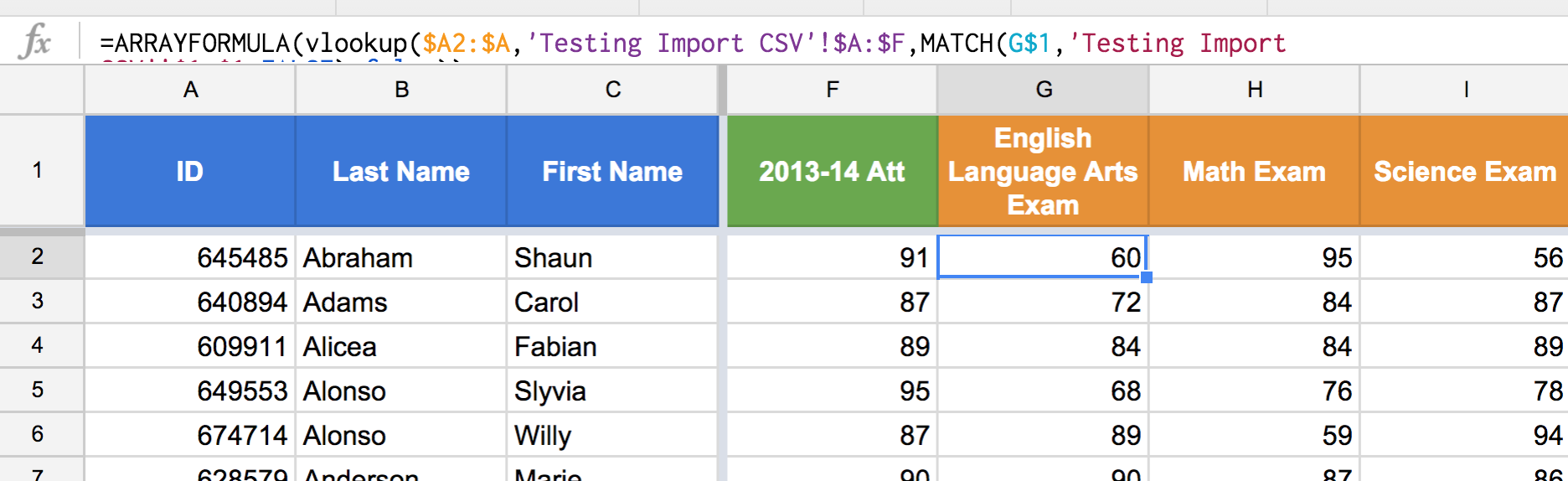Figure 22: And now our attendance data and exam data are side by side and we can look for students whose year to date attendance may be impacting their performance on specific exams.

### Conclusion

Here is an example of a finished product.

Once all of the ARRAYFORMULA(), VLOOKUP(), and MATCH() triumvirate functions have been properly copied and pasted with appropriate sheet references, you will have successfully merged data from multiple data core systems into a single parent sheet. Moving forward, replace the child Import CSV sheets using the same "File" and "Import" feature from the setup steps with updated imported data to keep the parent sheet up to date. Depending on the data, this update process can happen daily, weekly,  monthly, yearly, etc.

In my own experience as an Assistant Principal, I'm able to pull data from about a half dozen systems in under 20 minutes that gives me an incredibly complete picture of my students in a way that looking at each data point separately would completely obfuscate.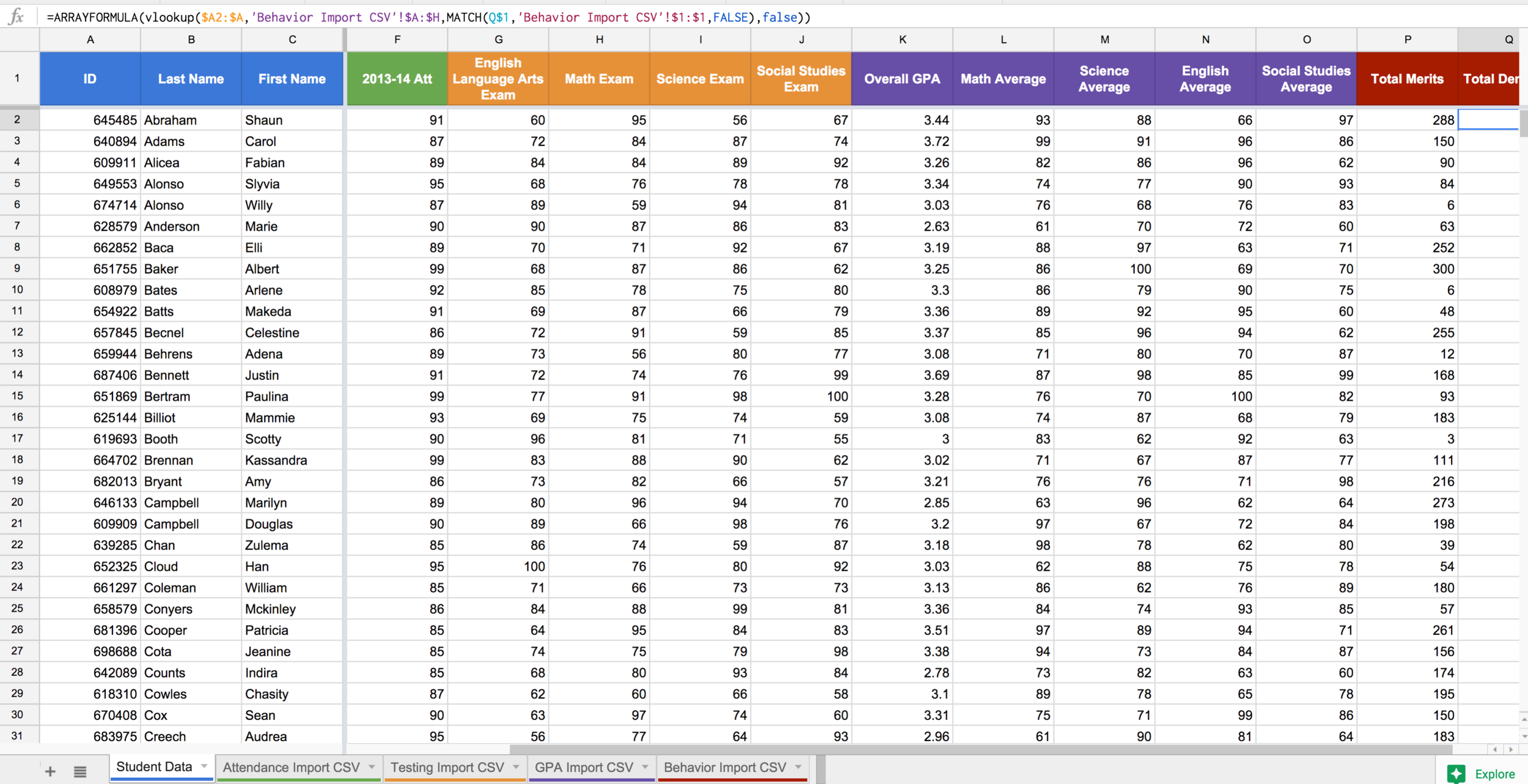Figure 23: The finished product!